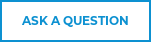# The Secret To Successful Thermistor Beta Calculations## What Do RT1, RT2, T1, and T2 Have In Common?

They are the main components of Thermistor Beta Calculations as it relates to temperature measurement, compensation, and control.

And if you are an engineer in an industry such as automotive or HVAC where things like coolant and air intake temperatures are crucial to the safety and performance of a vehicle or unit, chances are you will deal with beta calculations on a regular basis. Thus, when you need to replace a Thermistor, beta value calculations will become your best friend!## So Let’s Dig In. What Is Beta Value?

It is an indication of the shape of the curve that represents the relationship between the resistance and the temperature of a particular Thermistor. Therefore, you calculate the beta value to achieve the right characteristic at a given temperature vs the resistance for a specific application. The ability to calculate the beta value correctly is a vital step in the component selection process.

## What Does Resistance VS Temperature Mean In Relation To Thermistor Beta Calculations?

It’s simple. It is the characteristic or the curve of a component that best defines the resistance as a function of the temperature.

• Here is an example of how resistance vs temperature is used in a calculation. The Ohms represent the resistance that the Thermistor needs to obtain at a given temperature. 95Ω (Ohms) @ 38° C (Celsius)

## How Do You Calculate Beta?

• Thermistor Beta Calculations use a two-point calibration to calculate the resistance vs the temperature curve and take into consideration the required resistance at both temperature points.
• The Beta Value is measured in degrees Kelvin rather than Fahrenheit or Celsius, and it is calculated using the four components listed below.

1. RT1 = Resistance at Temperature 1
2. RT2 = Resistance at Temperature 2
3. T1 = Temperature 1 (K)
4. T2 = Temperature 2 (K)## Applying Thermistor Beta Calculations

In the example calculation below, the objective was to find a Thermistor with the specified resistance as part of a project to restore an antique tube radio.Example NTC Thermistor Beta Calculation

The beta calculation shown above resulted in the recommendation of an NTC Thermistor 2DB201K because 3307k closely relates to the shape of the resistance vs the plotted temperature.

Now that you have gained a better understanding of Thermistor Beta Calculations and how to apply them, we end this article with some trivia.

Pop Quiz: How did the letter K become the symbol for beta value?

We can thank William Thomson – also known as Lord Kelvin for that. He invented the “Kelvin Scale” Aka Kelvin in the 1800’s and it is still used in today’s temperature sensing calculations in conjunction with Fahrenheit and Celsius.

For more information, see our page on NTC Thermistor Beta or Explore Calculating the Temperature Coefficient of a Thermistor.

Need more help? Ask an Engineer.